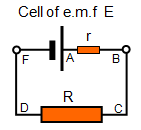## EMF and internal resistance

1. Explain what is meant by electromotive force (e.m.f.).

2. Explain what is meant by internal resistance.

3. Give one advantage of a battery having some internal resistance.4. Draw a diagram to how the potential varies around the circuit shown in the diagram.

5. Explain what happens to the output potential across the terminals of a cell with some internal resistance as the current from it is increased.

6. Explain what happens to the output potential across the terminals of a cell with some internal resistance when the external resistor (R in the diagram) is reduced.

7. A cell of e.m.f. 2.5 V and with an internal resistance of 0.2Ω is connected in turn to external resistors of 10Ω and then 1Ω. For each value of the external resistor calculate:
(a) the current in the circuit
(b) the potential difference across the terminals of the cell
(c) the power loss inside the cell

8. A digital voltmeter with resistance of 10 MΩ reads 1.30 V when connected across the terminals of a cell. When the same meter is connected across a resistor of 20Ω that has been connected in series with the cell the voltmeter reads 1.25V.
Explain the difference between these two readings and calculate:
(a) the current in the external resistor
(b) the internal resistance of the cell